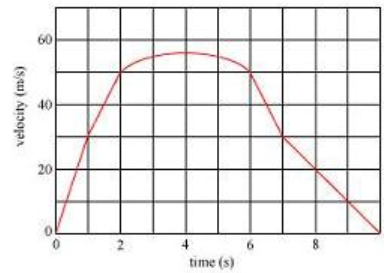# Problem: Find the minimum magnitude of the acceleration of the car.Express your answer in meters per second per second to the nearest integer.

###### FREE Expert Solution

The slope of the graph is the acceleration. From t = 0 s, the acceleration is positive. After t = 4 s, the acceleration changes direction and becomes negative.

79% (435 ratings)###### Problem DetailsFind the minimum magnitude of the acceleration of the car.
Express your answer in meters per second per second to the nearest integer.